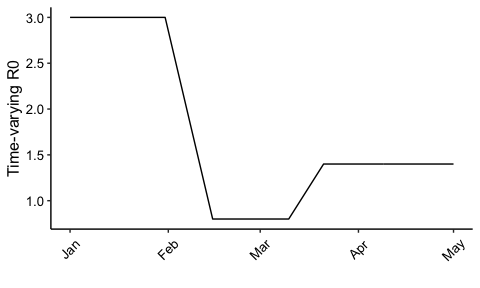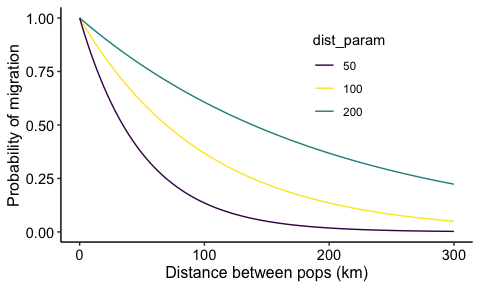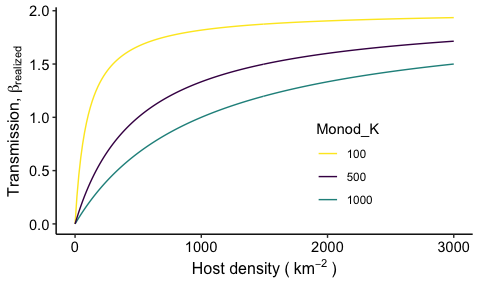# SPARSE-MOD Key Features

#### December 2020

library(tidyverse)
library(viridis)
library(lubridate)

## SPARSE-MOD: Overview and Key Features

SPARSE-MOD stands for SPAtial Resolution-SEnsitive Models of Outbreak Dynamics. Our goal with this R package is to offer a framework for simulating the dynamics of stochastic and spatially-explicit models of infectious disease. As we develop the package, our goal is to add more model structures and more user-control of the model dynamics. Our SPARSEMODr package offers several key features that should make it particularly relevant for pedogogical and practical use. See our COVID-19 model vignette and our SEIR model vignettefor detailed walk-throughs of how to run the model(s), to plot the output, and to simulate customized time-windows.

• Spatially explicit models that allow user-defined meta-populations1 characteristics and a customizable dispersal kernel (see below).

• Customizable process time-windows: The user controls how the time-varying R02 and the dynamics of host migration change during the simulated outbreak. We have created $$\texttt{time_window}$$ objects that allow users to simulate, for example, time periods over which public health or conservation interventions are implemented that can affect the effective contact between hosts or the movement of hosts among populations.

• Demographic stochasticity3 is built-in using a tau-leaping algorithm4. This captures the random transmission processes that are important early in outbreaks and especially in small host populations.

• Stochastic transmission is also built-in, allowing daily fluctuations in the transmission rate, which can help account for dynamics like super-spreading or super-shedding.

• The transmission process can be simulated as frequency-dependent (i.e., contact rates are invariable to population density) or density-dependent (i.e., contact rates depend on population density). For density-dependent transmission, we allow the user to custom-define a (non-)linear relationship between local host density and the transmission rate (see below).

• Models are coded in C++ and take advantage of Rcpp for rapid simulation of stochastic model trajectories across many focal populations.

## In-depth…

### Time windows

One of the benefits of the SPARSEMODr design is that the user can specify how certain parameters of the model change over time. In this particular example, we show how the time-varying R0 changes in a stepwise fashion due to ‘interventions’ and ‘release of interventions’. We assume that when a parameter value changes between two time windows, there is a linear change over the number of days in that window. In other words, the user specifies the value of the parameter acheived on the last day of the time window. Note, however, that the user is allowed to supply daily parameter values to avoid this linear-change assumption. Here we show an example of a pattern of time-varying R0 that the user might specify, and how the C++ code is essentially interpretting these values on the back-end.

# Set up the dates of change. 5 time windows
n_windows = 5
# Window intervals
start_dates = c(mdy("1-1-20"),  mdy("2-1-20"),  mdy("2-16-20"), mdy("3-11-20"), mdy("3-22-20"))
end_dates   = c(mdy("1-31-20"), mdy("2-15-20"), mdy("3-10-20"), mdy("3-21-20"), mdy("5-1-20"))
# Time-varying R0
changing_r0 = c(3.0,            0.8,            0.8,            1.4,            1.4)

#R0 sequence
r0_seq = NULL

r0_seq[1:(yday(end_dates) - yday(start_dates) + 1)] =
changing_r0

for(i in 2:n_windows){

r0_temp_seq = NULL
r0_temp = NULL

if(changing_r0[i] != changing_r0[i-1]){

r0_diff = changing_r0[i-1] - changing_r0[i]
n_days = yday(end_dates[i]) - yday(start_dates[i]) + 1
r0_slope = - r0_diff / n_days

for(j in 1:n_days){
r0_temp_seq[j] = changing_r0[i-1] + r0_slope*j
}

}else{
n_days = yday(end_dates[i]) - yday(start_dates[i]) + 1
r0_temp_seq = rep(changing_r0[i], times = n_days)
}

r0_seq = c(r0_seq, r0_temp_seq)

}

# Create a data frame for plotting
## Date sequence:
date_seq = seq.Date(start_dates, end_dates[n_windows], by = "1 day")
r0_seq_df = data.frame(r0_seq, date_seq)
date_breaks = seq(range(date_seq),
range(date_seq),
by = "1 month")

ggplot(r0_seq_df) +
geom_path(aes(x = date_seq, y = r0_seq)) +
scale_x_date(breaks = date_breaks, date_labels = "%b") +
labs(x="", y="Time-varying R0") +
# THEME
theme_classic()+
theme(
axis.text = element_text(size = 10, color = "black"),
axis.title = element_text(size = 12, color = "black"),
axis.text.x = element_text(angle = 45, vjust = 0.5)
)### Dispersal kernel

As we discuss in the documentation (see $$\texttt{?SPARSEMODr::Movement}$$), we allow migration between populations in the meta-population to affect local and regional transmission dynamics. For now, migration is determined by a simple dispersal kernel, although we are working on adding more customizable gravity kernels. The user can control the shape of this kernel with the $$\texttt{dist_param}$$ option, as follows: $p_{i,j} = \frac{1}{\text{exp}(d_{i,j} / \text{dist_param})},$ where $$p_{i,j}$$ is the probability of moving from population $$j$$ to population $$i$$ and $$d_{i,j}$$ is the euclidean distance between the two populations.

We can see how the $$\texttt{dist_param}$$ controls the probability below. In general, larger values of $$\texttt{dist_param}$$ make it more likely for hosts to travel farther distances.### Density-dependent Transmission

As we describe in the documentation (e.g., see $$\texttt{?SPARSEMODr::model_interface}$$), we allow the user to implement frequency-dependent or density-dependent (DD) transmission in the SPARSEMODr models. For DD transmission, the user can specify a (non-)linear Monod equation that describes the relationship between host population density and the transmission rate $$\beta$$ via the model’s (optional) parameter, $$\texttt{dd_trans_monod_k}$$. The Monod equation is: $\beta_{\text{realized}} = \beta_{\text{max}} \frac{\text{Dens}}{K + \text{Dens}},$ where $$\beta_{\text{max}}$$ is the maximum possible transmission rate across all densities, $$\texttt{Dens}$$ is the density of the focal host population, and $$K$$ is a constant that controls the effect of density on the transmission rate and is user-controlled by specifying $$\texttt{dd_trans_monod_k}$$. More specifically, $$K$$ is the half-velocity constant at which point $$\beta_{\text{realized}}/\beta_{\text{max}} = 0.5$$.

We can see how $$\texttt{dd_trans_monod_k}$$ controls the transmission rate below. In general, larger values of $$\texttt{dd_trans_monod_k}$$ mean that transmission rate is more strongly limited by population density.1. A set of distinct, focal populations that are connected by migration

2. Also known as the effective reproduction number or the instantaneous R0 (Rt)

3. The effects of probabilistic events that befall a population and that can affect epidemic trajectories.

4. Models are based off of differential equation models, but we use a tau-leaping algorithm - in the Gillespie family - to simulate the model one day at a time.